# NCERT Solutions for Class 8 Maths Chapter 1 Rational Numbers Ex 1.2

NCERT Solutions for Class 8 Maths Chapter 1 Rational Numbers Exercise 1.2

Ex 1.2 Class 8 Maths Question 1.
Represent these numbers on the number line
(i) $$\frac { 7 }{ 4 }$$
(ii) $$\frac { -5 }{ 6 }$$
Solution:
(i) $$\frac { 7 }{ 4 }$$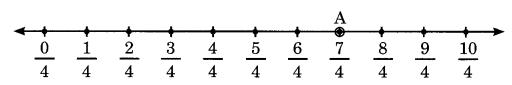Here, point A represents $$\frac { 7 }{ 4 }$$ on the number line.
(ii) $$\frac { -5 }{ 6 }$$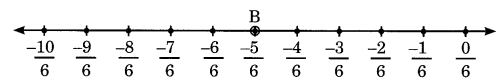Here, point B represents $$\frac { -5 }{ 6 }$$ on the number line.

Ex 1.2 Class 8 Maths Question 2.
Represent $$\frac { -2 }{ 11 }$$, $$\frac { -5 }{ 11 }$$ , $$\frac { -9 }{ 11 }$$ on a number line.
Solution:
We have $$\frac { -2 }{ 11 }$$, $$\frac { -5 }{ 11 }$$ and $$\frac { -9 }{ 11 }$$Here, point A represents $$\frac { -2 }{ 11 }$$ , point B represents $$\frac { -5 }{ 11 }$$, point C represents $$\frac { -9 }{ 11 }$$

Ex 1.2 Class 8 Maths Question 3.
Write five rational numbers which are smaller than 2.
Solution:
Required five rational numbers smaller than 2 are
1, 0, $$\frac { 1 }{ 2 }$$, $$\frac { 1 }{ 3 }$$ and $$\frac { 1 }{ 4 }$$

Ex 1.2 Class 8 Maths Question 4.
Find ten rational numbers between $$\frac { -2 }{ 5 }$$ and $$\frac { 1 }{ 2 }$$.
Solution: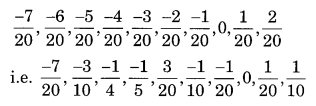Ex 1.2 Class 8 Maths Question 5.
Find five rational numbers between
(i) $$\frac { 2 }{ 3 }$$ and $$\frac { 4 }{ 5 }$$
(ii) $$\frac { -3 }{ 2 }$$ and $$\frac { 5 }{ 3 }$$
(iii) $$\frac { 1 }{ 4 }$$ and $$\frac { 1 }{ 2 }$$
Solution: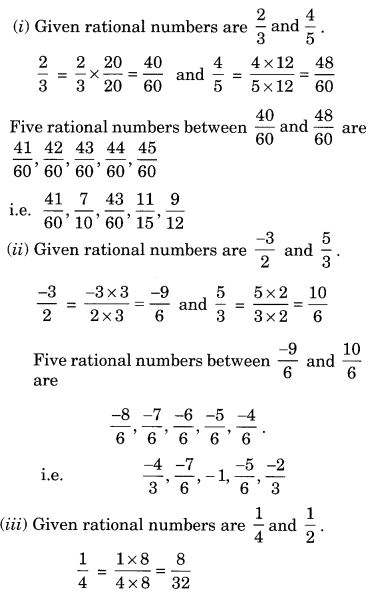Ex 1.2 Class 8 Maths Question 6.
Write five rational numbers greater than -2.
Solution:
Required rational numbers greater than -2 are -1, 0, $$\frac { 1 }{ 2 }$$, $$\frac { 3 }{ 4 }$$ , 1.

Ex 1.2 Class 8 Maths Question 7.
Find ten rational numbers between $$\frac { 3 }{ 5 }$$ and $$\frac { 3 }{ 4 }$$.
Solution:
Given rational numbers are $$\frac { 3 }{ 5 }$$ and $$\frac { 3 }{ 4 }$$.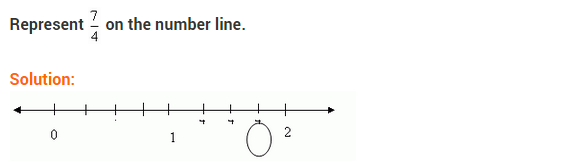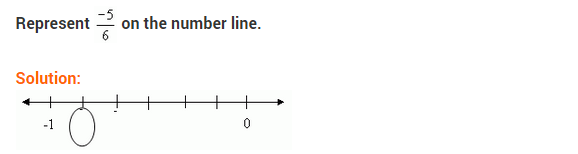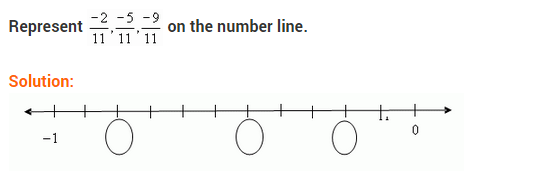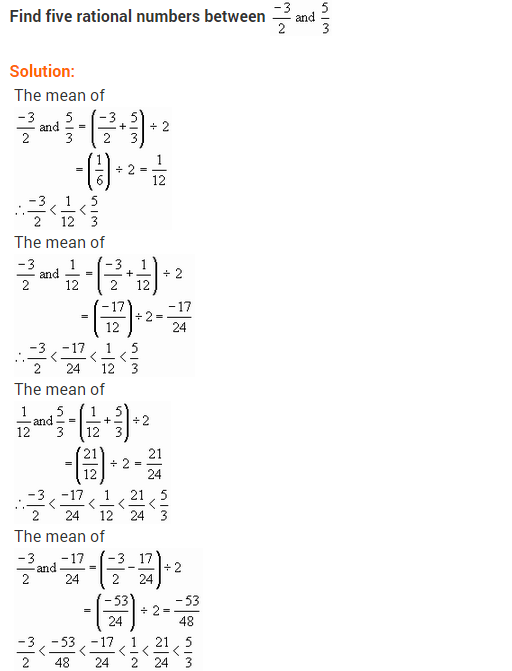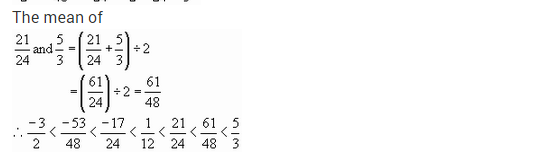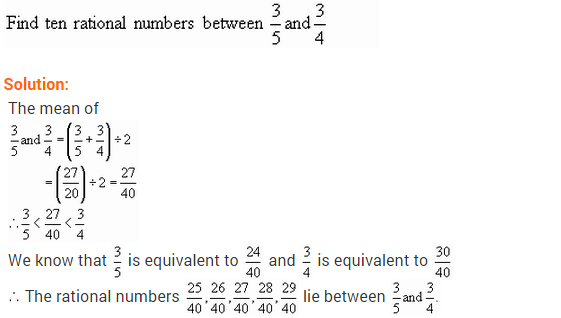+